# Introduction

In the paper, the authors introduce a new unsupervised deep learning method — Invariant Information Clustering (IIC). IIC directly trains a randomly initialized neural network into a classification function, end-to-end and without any labels. It involves a simple objective function, which is the mutual information between the function’s classifications for paired data samples.

IIC can be applied to image clustering as well as image segmentation. In this project, we reproduce the segmentation part. In the image segmentation process, we do some transformations(e.g. flipping, colorjitting) to each image and add displacements to each pixel.

# Prepare data

In the reproduction project, we use Potsdam dataset in training. The dataset consists of 5400 different satellite landscape images. There are two main reasons for us to choose this dataset. One is that the size of the Potsdam dataset is relatively small, which means less computation required. Another reason is that there’s no need to select images out of the dataset by the percentage of “stuff pixel” like Cocostuff. Before applying the data to the model, we do some preparation work.

The Potsdam dataset is in the format of .mat files. It is divided into a training set and ground truth set. For training set, each picture file is a 200*200*4 matrix, which represents 200*200 pixels and R, G, B, IR value for each pixel. The ground truth set consists of 200*200*1-dimension .mat files, which represents 200*200 pixels with label 1–6 on each pixel.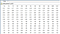Part of data in a single img file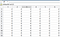Part of data in a single gt file

For data augmentation, we apply horizontal flip to each picture in the training set by flipping along its symmetry axis. Also, we apply the colorjitter function to the training set. All four parameters (brightness, contrast, saturation and hue) are set to 0.1 according to their implementation.

# Model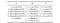Model selection

Network architectures given in supplement material. By segmentation, VGG network C, which is shown in Figure 3 is used.

We use the model created in the original IIC, which can be found in SegmentationNet10a class in the original code. As some parameters such as the kernel size and stride are already predefined and hardcoded in the code, what we need to do is correctly define the input channels (4)and output channels (3 or 6).

In the paper and supplement material they mentioned the benefit of overclustering, we follow this instruction and use SegmentationNet10aTwoHead class, it is a network with two output layer, one with the output size equals the amount of class label (3 for Potsdam 3 and 6 for Potsdam), the other with 3 times larger output size. In this case we can calculate 2 losses, we average the two losses.

# Our original implementation

In the beginning, we tried to follow the IIC paper’s step and reproduced the code strictly by the equations presented in the paper. However, it is too slow to run on the full Potsdam data set. But anyway, as a part of our project, it is still worth explaining our original implementation.

In the process, we performed pixel-by-pixel comparison by computing the outer product of two vectors into a matrix, and then took the average. After that, we computed such a matrix for each image in one batch and took the average. Since each batch consisted of 2 transformation — flipping and colorjitting, so the same process should be done for each transformation. Then we calculated the loss for the matrix we have so far. This procedure applied to function 5 in the IIC paper, and the way of computing mutual information is described in function 3 of the paper.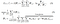Equations we used originally to calculate loss function

The goal is to maximize the information between each pixel Φu(xi) and the patch label [g−1Φ(gxi)]u+t of its transformed neighbour patch, in expectation over images i = 1, . . . , n, patches u ∈ Ω within each image, and perturbations g ∈ G. Information is in turn averaged over all neighbour displacements t ∈ T . We assumed the displacement process was moving one pixel to 8 directions (E, W, S, N, NE, NW, SE, SW), so loss calculation needs to be done for each of them. 

While training, we first implemented a small demo with 16 images and ran it on Google Colab, and then ran the whole dataset. However, due to the large amount of computation and storage, The RAM got full immediately, which led to a decrease of computing speed. It is approximated that training the whole dataset may take a few weeks.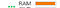Full RAM

Even worse, the cuda failed to work properly when we ran the model on the whole dataset. Normally, the batch size should be 60. However, the cuda ran out of memory when the batch size was larger than 10. So we finally had to change the computing method and applied the loss function in the authors’ code.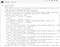Cuda out of memory

We adapted the author’s loss function called IID_segmentation_loss, which takes the output (class probability distribution) of two batches (one for the original images and the other for the transformed images) as input and calculates the information as output. We can not understand how this function works exactly since it is implemented in a smart and complex way, with this function we don’t suffer from the full RAM problem above anymore. Besides, the loop through all the transformations and displacements seems to be done in this one function, but we do not understand how exactly and question whether it implements equation 5 strictly. These confusions were also the reason why we were uncomfortable to use their loss function directly at the beginning, and only adapt to it in the end.

Although the RAM problem has been solved, the problem with batch size still exists. We can not run size 75 for Potsdam-6 (or 60 for Potsdam- 3), instead, the maximum batch size we can run without getting “Cuda out of memory error” is 20. In their experiments, they run thousands of epochs, which is also what we are unable to, seeing the time we have left.

Finally, we decided to limit the batch size to 20. However, something unexpected happened. The GPU of Google colab always stop working in the midway of our validation process, which directly led to failure to calculate the final accuracy. The most probable reason might be system failure.

# Result

Unfortunately, we are not able to fully reproduce the paper. It is partly due to hardware issues like failure of cuda and Google colab, and also because the original code provided by the authors of IIC paper is quite messy. However, the good thing is that our loss function goes on the right way towards optimization. The figure below shows the trend of loss function. Since the loss is based on mutual information, the larger absolute value this loss reaches, the better effect it could be.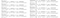Trend of loss function

In future days, we plan to find out the problems and try to fully reproduce the paper if time is available.

# Task distribution

Dina Chen

1. Getting Potsdam data ready as input to the model

2. Data transformation: flip and color jitter

3. Reproduce equation 5 and equation 3 from the paper (calculate the loss from output of model)

4. Adapt model and loss function from the original code

5. Run experiments

Zhiyi Wang

1. Study and pre-process the datasets (both CocoStuff and Potsdam)

2. Run our model on Potsdam3 dataset

3. Write validation function to calculate accuracy based on ground truth and the trained result

# References

 Ji X, Henriques J F, Vedaldi A. Invariant information clustering for unsupervised image classification and segmentation[C]//Proceedings of the IEEE/CVF International Conference on Computer Vision. 2019: 9865–9874.

 Potsdam Dataset

https://www.robots.ox.ac.uk/~xuji/datasets/Potsdam.tar.gz

# Appendix

Github link of our reproduction code:

https://github.com/DinaChen/IIC-segmentation

Github link of the original authors’ code:

https://github.com/xu-ji/IIC